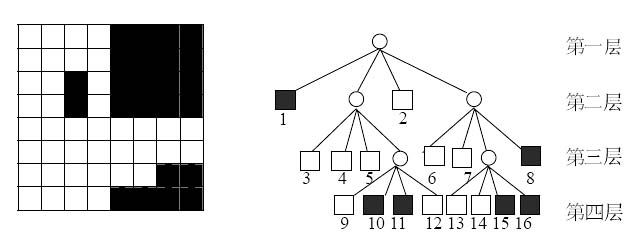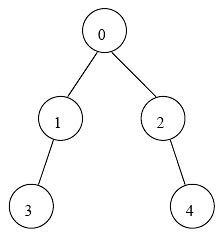HNFMS一个初二蒟蒻OIerの实验室

# 2019年10月3日测试解题报告

### T1 高中运动会(match)

[问题描述]

[输入格式]

[输出格式]

``````3
12 16 20``````

[样例输出 1]

``4``

``````#include<bits/stdc++.h>
#define ini(...) scanf("%d",__VA_ARGS__)
#define oui(...) printf("%d",__VA_ARGS__)
#define in(...) scanf(__VA_ARGS__)
#define ou(...) printf(__VA_ARGS__)
#define F(i,a,b) for(int (i)=(a);(i)<=(b);(i)=-~(i))
#define DF(i,a,b) for(int (i)=(a);(i)>=(b);(i)--)
#define fin(...) freopen(__VA_ARGS__,"r",stdin)
#define fout(...) freopen(__VA_ARGS__,"w",stdout)
int n,gcd,temp;

int main()
{
fin("match.in");
fout("match.out");
ini(&n);
F(i,1,n)
{
ini(&temp);
gcd=(i==1?temp:std::__gcd(gcd,temp));
}
oui(gcd);ou("\n");
return 0;
}``````

### T2 订货( orders )

[问题描述]

[输入格式]

[输出格式]

[样例输入]

bbjd

[样例输出]

bbdj
bbjd
bdbj
bdjb
bjbd
bjdb
dbbj
dbjb
djbb
jbbd
jbdb
jdbb

``````#include<bits/stdc++.h>
#define ini(...) scanf("%d",__VA_ARGS__)
#define oui(...) printf("%d",__VA_ARGS__)
#define in(...) scanf(__VA_ARGS__)
#define ou(...) printf(__VA_ARGS__)
#define F(i,a,b) for(int (i)=(a);(i)<=(b);(i)=-~(i))
#define DF(i,a,b) for(int (i)=(a);(i)>=(b);(i)--)
#define fin(...) freopen(__VA_ARGS__,"r",stdin)
#define fout(...) freopen(__VA_ARGS__,"w",stdout)
using namespace std;
char ch,bak1;
int flag=1;

int main()
{
fin("orders.in");
fout("orders.out");
in("%s",&ch);
int len=strlen(ch);
sort(ch,ch+len);
while(1)
{
if(flag!=1)
{
next_permutation(ch,ch+len);
if(strcmp(ch,bak1)==0)break;
}
else
{
memcpy(bak1,ch,sizeof(ch));
}
ou("%s\n",ch);
flag=0;
}
return 0;
}``````

### T3 黑白影像 ( blackandwhite )

[问题描述]（左：图一 右：图二）

g b g w w w g w b b w w g w w g w w b b b

[输入格式]

[输出格式]

[样例输入1]

4
0000
0100
0011
0011

[样例输出1]

g w g w w w b w b

``````#include<bits/stdc++.h>
#define ini(...) scanf("%d",__VA_ARGS__)
#define oui(...) printf("%d",__VA_ARGS__)
#define in(...) scanf(__VA_ARGS__)
#define ou(...) printf(__VA_ARGS__)
#define F(i,a,b) for(int (i)=(a);(i)<=(b);(i)=-~(i))
#define DF(i,a,b) for(int (i)=(a);(i)>=(b);(i)--)
#define fin(...) freopen(__VA_ARGS__,"r",stdin)
#define fout(...) freopen(__VA_ARGS__,"w",stdout)
using namespace std;
int __map;
int n;

char check(int x,int y,int l,int r)
{
bool white=false,black=false;
F(i,x,y)
{
F(j,l,r)
{
if(__map[i][j]==1)
black=true;
else white=true;
if(black&&white)goto bk;
}
}
bk:
if(white&&black)return 'g';
else if(white)return 'w';
else return 'b';
}

int dfs(int x,int y,int l,int r)
{
//第一象限
char temp=check(x,(y+x)>>1,((l+r)>>1)+1,r);
if(temp=='g')
{
ou("g ");
dfs(x,(y+x)>>1,((l+r)>>1)+1,r);
}
else ou("%c ",temp);

//第二象限
temp=check(x,(y+x)>>1,l,(r+l)>>1);
if(temp=='g')
{
ou("g ");
dfs(x,(y+x)>>1,l,(r+l)>>1);
}
else ou("%c ",temp);

//第三象限
temp=check(((y+x)>>1)+1,y,l,(r+l)>>1);
if(temp=='g')
{
ou("g ");
dfs(((y+x)>>1)+1,y,l,(r+l)>>1);
}
else ou("%c ",temp);

//第四象限
temp=check(((y+x)>>1)+1,y,((r+l)>>1)+1,r);
if(temp=='g')
{
ou("g ");
dfs(((y+x)>>1)+1,y,((r+l)>>1)+1,r);
}
else ou("%c ",temp);
}

int main()
{
fin("blackandwhite.in");
fout("blackandwhite.out");
ini(&n);
F(i,1,n)
{
F(j,1,n)
{
in("%1d",&__map[i][j]);
}
}
char temp1=check(1,n,1,n);
if(temp1=='g')
{
ou("g ");
dfs(1,n,1,n);
}
else ou("%c ",temp1);
ou("\n");
return 0;
}``````

### T4 银河帝国旅行社( galaxy )

[问题描述][输入格式]

[输出格式]

[样例输入1]

``````5
1 2 -1        //首都（0号星）的从属星是1号星与2号星
3 -1            //1号星的从属星是3号星
4 -1            //2号星的从属星是4号星
-1              //3号星没有从属星
-1              //4号星没有从属星``````

[样例输出1]

``4``

``````#include<bits/stdc++.h>
#define ini(...) scanf("%d",__VA_ARGS__)
#define oui(...) printf("%d",__VA_ARGS__)
#define in(...) scanf(__VA_ARGS__)
#define ou(...) printf(__VA_ARGS__)
#define F(i,a,b) for(int (i)=(a);(i)<=(b);(i)=-~(i))
#define DF(i,a,b) for(int (i)=(a);(i)>=(b);(i)--)
#define fin(...) freopen(__VA_ARGS__,"r",stdin)
#define fout(...) freopen(__VA_ARGS__,"w",stdout)
std::vector<int> a;
int n,x,s,mx,random,xlong;
bool lock;

void dfs(int l){
F(i,0,a[l].size()-1)
if(!lock[a[l][i]]){
lock[a[l][i]]=1;
s[a[l][i]]=s[l]+1;
dfs(a[l][i]);
}
}

int calc(int v){
memset(lock,0,sizeof(lock));
s[v]=mx=0;
dfs(v);
F(i,0,n-1)
mx=std::max(mx,s[i]);
F(i,0,n-1)
if(s[i]==mx)
return i;
}

int main()
{
fin("galaxy.in");
fout("galaxy.out");
ini(&n);
F(i,0,n-1)
{
while(ini(&x)!=EOF){
if(x<0) break;
a[x].push_back(i);
a[i].push_back(x);
}
}
random=calc(0);
xlong=calc(random);
oui(mx);ou("\n");
return 0;
}
``````

Title - Artist
0:00